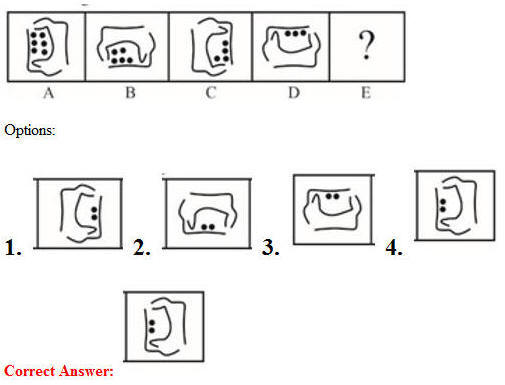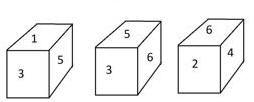# 10 July 2019 – Afternoon Shift (Reasoning)

SSC CHSL (Tier – 1) Online Exam Paper – 2018 “held on 10 July 2019”

Afternoon Shift (Reasoning)

Q1. Select the word-pair in which the two words are related in the same way as are the two words in the following word-pair.
Mumbai : Capital

Options:

1. Yen : Currency
2. Delhi : Taj mahal
3. New york : China
4. Hill : High

Q2. In a code language, ROCKET is written as TEKROC. How will DRIVER be written as in that language?

Options:

1. REUDRI
2. REVDRJ
3. RFVDRI
4. REVDRI

Q3. A paper is folded and cut as shown below. How will it appear when unfolded?Q4. Select the option that is related to the third letter-pair in the same way as the second letter-pair is related to the first letter-pair.
GI : KN : : PQ : ?

Options:

1. TX
2. TV
3. VT
4. UV

Q5. Three of the following four numbers are alike in a certain way and one is different. Pick the number that is different from the rest.

Options:

1. 259
2. 639
3. 459
4. 189

Q6. Which number will replace the question mark (?) in the following series?
12, 22, 42, 72, ?

Options:

1. 102
2. 112
3. 92
4. 122

Q7. Select the number-pair in which the two numbers are related in the same way as are the two numbers of the following number-pair.
8 : 72

Options:

1. 11 : 132
2. 12 : 144
3. 13 : 156
4. 15 : 625

Q8. If EAST is coded as 180 and NORTH is coded as 375, then how will SOUTH be coded as?

Options:

1. 83
2. 332
3. 625
4. 415

Q9. Select the Answer Fig. that will come next in the following Problem Fig. series.Q10. If it was a Sunday on 1 January 2017, what was the day of the week on 31 December 2017?

Options:

1. Tuesday
2. Sunday
3. Friday
4. Monday

Q11. S is son of M. R is the father of Q and A. A is the daughter of M. How is A related to S?

Options:

1. Sister
2. Daughter
3. Aunt
4. Mother

Q12. Three different positions of a dice are shown below. Which number appears on the face opposite the number 6?Options:

1. 1
2. 5
3. 4
4. 3

Q13. Which letter-pair will replace the question mark (?) in the following series?
HE, ? , NK, TQ, BY

Options:

1. IK
2. IG
3. JG
4. JH

Q14. Select the Venn diagram that best illustrates the relationship between the following classes.
Flowers, Roses, YellowOptions:

1.
2.
3.
4.

Q15. Three of the following four letter-clusters are alike in a certain way and one is different. Pick the odd one out.

Options:

1. DGL
2. KOS
3. BFJ
4. MQU

Q16. Select the set in which the numbers are related in the same way as are the numbers of the following set.
(16, 64, 320)

Options:

1. (15, 45, 180)
2. (10, 40, 200)
3. (25, 75, 125)
4. (15, 60, 310)

Q17. How many squares are present in the following figure:Options:

1. 10
2. 12
3. 15
4. 16

Q18. Three statements are given, followed by three conclusions numbered I, II and III. Assuming the statements to be true, even if they seem to be at variance with commonly known facts, decide which of the conclusions logically follow(s) from the statements.

Statements:
All hens are eggs.
No birds are eggs.
All eggs are lions.

Conclusions:
I. All hens are lions.
II. Some lions are hens.
III. No eggs are birds.

Options:

1. Only conclusions I and II follow.
2. Either conclusion II or III follows.
3. All the conclusions follow.
4. Only conclusion I follows.

Correct Answer: All the conclusions follow

Q19. Which two signs should be interchanged in the following equation to make it correct?
35 + 7 – 5 × 2 ÷ 25 = 20

Options:

1. + and ÷
2. × and –
3. × and ÷
4. + and –

Q20. Three of the following four words are alike in a certain way and one is different.
Pick the odd word out.

Options:

1. Duster
2. Stationery
3. Blackboard
4. Chalk

Q21. Select the correct mirror image of the given figure is placed to the left of the figure.Q22. Arrange the following words in a logical sequence as they would appear in the English dictionary.

1. Jacklight
2. Jacket
4. Joker
5. Jampacked

Options:

1. 2, 3, 1, 4, 5
2. 3, 1, 2, 5, 4
3. 2, 1, 3, 5, 4
4. 1, 2, 3, 4, 5

Correct Answer: 2, 1, 3, 5, 4

Q23. ‘HONEY’ is related to ‘BEE’ in the same way as ‘EGG’ is related to ‘________’.

Options:

1. YOLK
2. OOLOGY
3. BIRD
4. LARVA

Q24. Select the option in which the given figure is embedded.Q25. Select the set in which the numbers are related in the same way as are the numbers of the following set.
(15, 49, 151)

Options:

1. (5, 19, 61)
2. (25, 79, 137)
3. (17, 55, 155)
4. (6, 18, 37)